Courses

# Test: Design Of Joints (Level - 2)

## 20 Questions MCQ Test Machine Design | Test: Design Of Joints (Level - 2)

Description
This mock test of Test: Design Of Joints (Level - 2) for Mechanical Engineering helps you for every Mechanical Engineering entrance exam. This contains 20 Multiple Choice Questions for Mechanical Engineering Test: Design Of Joints (Level - 2) (mcq) to study with solutions a complete question bank. The solved questions answers in this Test: Design Of Joints (Level - 2) quiz give you a good mix of easy questions and tough questions. Mechanical Engineering students definitely take this Test: Design Of Joints (Level - 2) exercise for a better result in the exam. You can find other Test: Design Of Joints (Level - 2) extra questions, long questions & short questions for Mechanical Engineering on EduRev as well by searching above.
QUESTION: 1

### A double fillet welded joint with parallel fillet weld of length L and leg B is subjected to a tensile force P. Assuming uniform stress distribution, the shear stress in the weld is given by

Solution: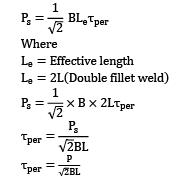Where

Le = Effective length

Le = 2L(Double fillet weld).

QUESTION: 2

### A circular solid rod of diameter d welded to a rigid flat plate by a circular fillet weld of throat thickness t is subjected to a twisting moment T. The maximum shear stress induced in the weld is

Solution: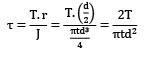QUESTION: 3

### Pre loading of bolts in structural joints and pressure vessels subjected to fluctuating loads?

Solution:

Pre-loading of bolts will help in following

-Increasing the stiffness of the bolt

-Securely tightening the mating parts

-If above two things will be there, so it will also avoid leakage.

-So based upon the options, the most correct option is B.

QUESTION: 4

In transverse fillet weld, the size of the weld is equal to

Solution:

0.707S = t

S = 1.414 t

S =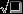t

Where S = weld size

t = throat thicknes

QUESTION: 5

The permissible stress in a fillet weld is 100 N/mm2 . The fillet weld has equal leg lengths of 15 mm each. The allowable shearing load on weldment per cm length of the weld is

Solution:

Strength of weld P = 0.707 hlτper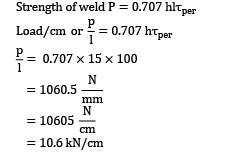= 10.6 kN/cm

QUESTION: 6

Tearing efficiency of a double riveted lap joint having a pitch of 60 mm and rivet hole diameter of 18 mm is

Solution: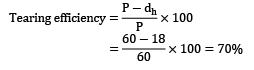QUESTION: 7

Two metal plates of thickness ‘t’ and width ‘w’ are joined by a fillet weld of as shown in given figure.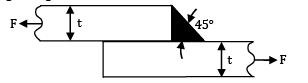When subjected to a pulling force ‘F’, the stress induced in the weld will be

Solution:

Strength of a fillet weld is given by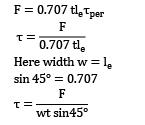Here width w = le

Sin 45o = 0.707QUESTION: 8

In the welded joint shown in the given figure, if the weld at B has thicker fillets than that at A, then the load carrying capacity P, of the joint will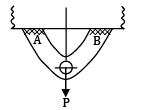Solution:

The load carrying capacity means the maximum load which a member can sustain without a failure. So because of load P the weaker section will fail first. So thicker fillet at B will have no effect on the load carrying capacity

QUESTION: 9

A circular rod of diameter d is welded to a flat plate along its circumference by fillet weld of thickness t. Assuming as the allowable shear stress for the weld material, what is the value of the safe torque that can be transmitted?Options~

(A)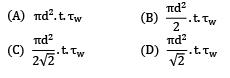(B)(C)(D)Solution:

Shear stress = τw

Shear force = τw x πdt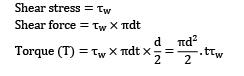QUESTION: 10

A single parallel fillet weld of total length L and weld size h subjected to a tensile load P, will have what design stress?

Solution: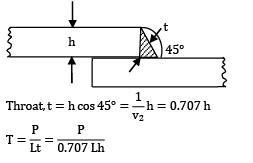QUESTION: 11

How can shock absorbing capacity of a bolt be increased?

Solution:

Making shank diameter equal to the core diameter of thread will increase the strain energy stored in the bolt which will increase the shock absorbing capacity of a bolt.

QUESTION: 12

By reducing the shank diameter equal to the root diameter the impact strength of bolts

Solution:

Reducing shank diameter equal to the root diameter will increase the strain energy of the bolt. And increase in strain energy will improve the impact strength of the bolts.

*Answer can only contain numeric values
QUESTION: 13

Shown in the figure is a bracket attached to a channel by means of 5 identical rivets. The maximum load on most heavily stressed rivet is __________ kN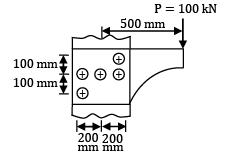Solution:

Primary shear force on each rivet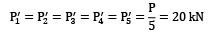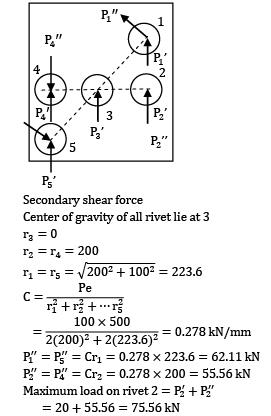Secondary shear force

Centre of gravity of all rivet lie at 3.

r3 = 0

r2 = r4 = 200P1” = P5” = Cr1 = 0.278 x 223.6 = 62.11 kN

P2” = P4” = Cr2 = 0.278 x 200 = 55.56 kN

Maximum load on rivet 2 = P2’ + P2

= 20 + 55.56 = 75.56 kN

Maximum load on rivet 1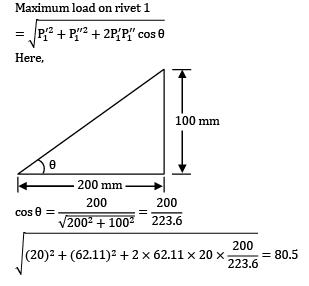Here,*Answer can only contain numeric values
QUESTION: 14

What is the diameter of the hole that must be drilled in a M50 (core diameter mm) bolt such the bolt becomes of uniform strengths is __________ mm?

Solution: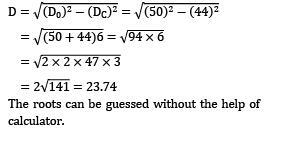=== 2= 23.74

The roots can be guessed without the help of calculator:

*Answer can only contain numeric values
QUESTION: 15

A shaft of diameter D is welded to a plate as shown in figure. A torque T is applied at the free end. If the maximum shear stress produced is given by . Where h is the leg length of weld, then the value of n is __________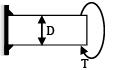Solution: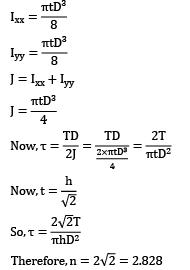J = lxx + IyyTherefore,n = 2*Answer can only contain numeric values
QUESTION: 16

A component is attached to a horizontal column by means of three identical rivets as shown in figure. The maximum permissible shear stress for the rivets is N mm . The magnitude of maximum force is __________ kN.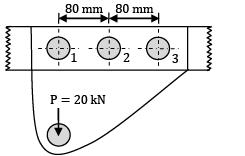Solution: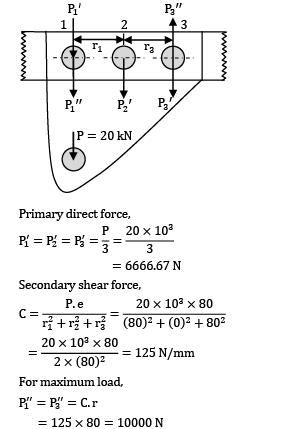Primary direct force,= 6666.67 N

Secondary shear force,For maximum load,

P1” = P3” = c.r

= 125 x 80 = 10000N

Resultant shear force,

P = P1’ + P1” = 6666.67 + 10000

= 16666.67 N

= 16.7 kN

QUESTION: 17

A plate is attached using 4 rivets as shown in the figure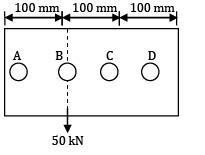If the maximum allowable shear stress is 80 MPa, then what will be the minimum diameter of rivets required (in mm)?

Solution:

Direct shear force,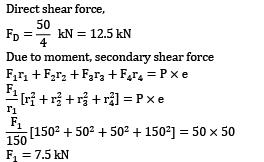Due to moment, secondary shear force

F1r1 + F2r2 + F3r3 + F4r4 = P x eF1 = 7.5 kN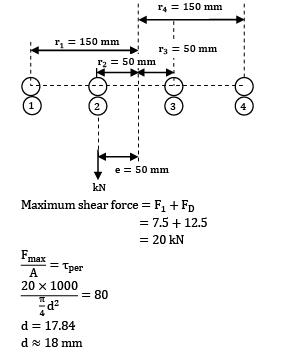Maximum shear force = F1 + FD\

= 7.5 + 12.5

= 20 kNd = 17.84

d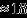mm

QUESTION: 18

The circumferential joint of a boiler of diameter 1 m is made of lap joint with rivet diameter of 20 mm. If the allowable shear stress for the material of the rivet is 50 MPa and the boiler has to withstand an internal pressure of 2.5 MPa, then the minimum number of rivets required to prevent the failure of the joint is

Solution: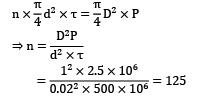QUESTION: 19

A shaft of 100 mm diameter is welded to a flat plate by a fillet weld of weld size 16 mm as shown in figure. If the allowable shear stress for the weld material is 50 MPa, the maximum torque the shaft can withstand to avoid weld failure is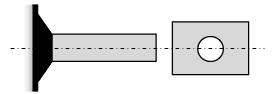Solution: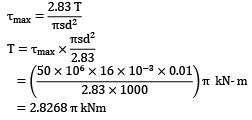= 2.8268π kNm

QUESTION: 20

Two plates are joined together by means of single transverse and double parallel fillet welds as shown in the figure given below. If the size of fillet is 5 mm and allowable shear load per mm is 300 N, what is the approximate length of each parallel fillet?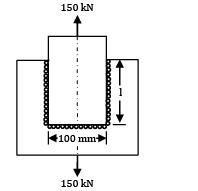Solution:

300 x (100 + 2I) = 15000 or I = 200